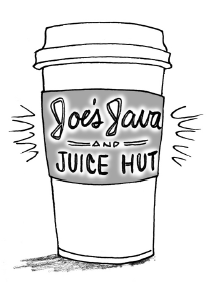### Home > INT2 > Chapter 9 > Lesson 9.4.1 > Problem9-145

9-145.Of the $63$ drinks at Joe’s Java and Juice Hut, $42$ contain coffee and $21$ contain dairy products. If Alexei randomly chooses a drink, what is the probability of getting a drink with both coffee and a dairy product? What is the probability of getting neither coffee nor a dairy product? Assume that choosing a coffee drink is independent of choosing a dairy-product drink. See if you can answer these questions without first making a two-way table.

If events A and B are independent, the P(A and B) = P(A) · P(B).

$\text{If P(coffee) = } \frac{42}{63} \text{, what is P(no coffee)? }$

$\text{If P(dairy) = } \frac{ 21}{63} \text{, what is P(no dairy)?}$